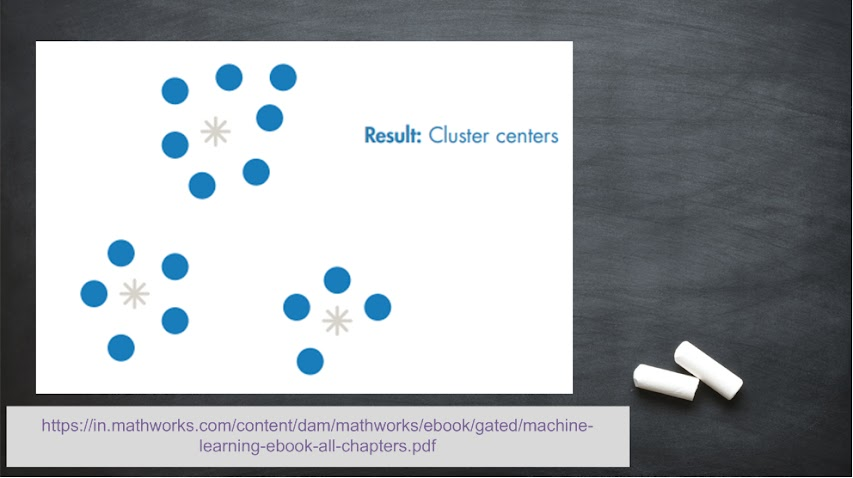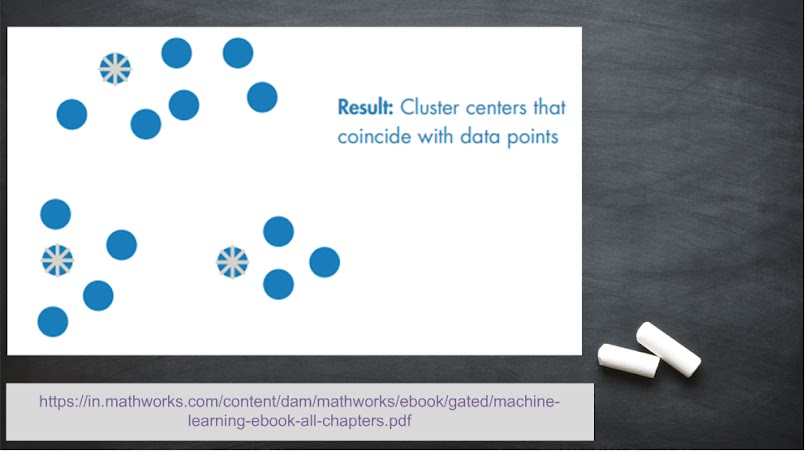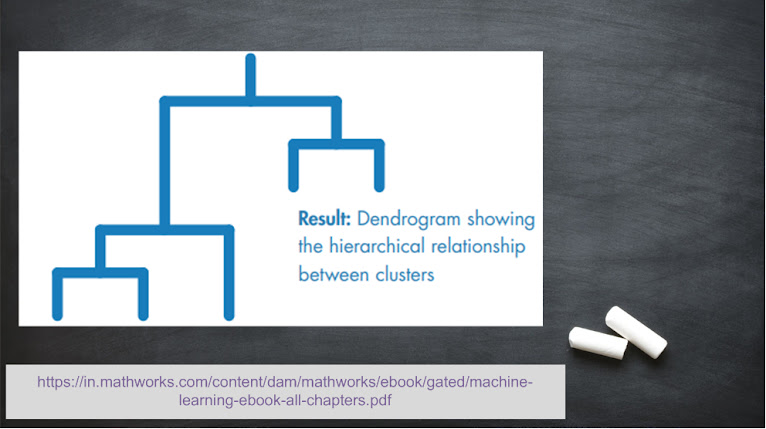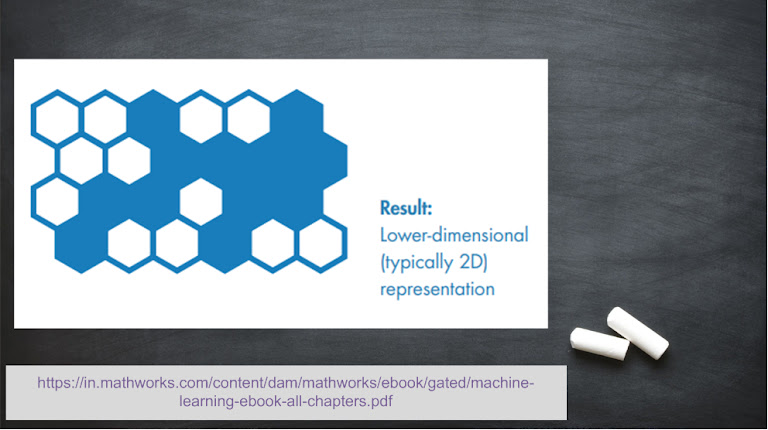Hello Readers!! Hope you understood classification and regression algorithms. Now we will proceed to clustering techniques. In simple English, cluster is group of objects of same or different classes. Now the thing is, we have a large number of data values in the available dataset. What we want is to re-group those data values such that similar data values lie in same cluster while distinct data values lie in different clusters.

Clustering is broadly classified in two categories:

• Hard Clustering:
When each data value belongs to only one cluster.
• Soft Clustering:

When each data value can belong to more than one cluster.

Various hard clustering-based techniques implemented in machine learning-based applications are as follows:

#### k-Means

In this technique, the dataset is divided into ‘k’ number of mutually exclusive clusters. The centre of each cluster is estimated. Whenever the model is tested for any data value, the distance of that data value is calculated from the centre of all the clusters, and it is placed in the cluster with nearest centre. Key points are as follows:
• Used for fast clustering of large datasets.
• Used when number of clusters is known.#### k-Medoids

Unlike k-Means technique, the centre of a cluster coincides with a data value which is present in that cluster. Key points are as follows:
• Used for fast clustering of large datasets.
• Used when number of clusters is known.
• Used for scaling large datasets.#### Hierarchical Clustering

In this technique, the similarity between pairs of data values is studied such that the similar data values are grouped together. The term ‘hierarchy’ means either we can approach in top-down manner (divisive manner) or in bottom-up manner (agglomerative manner). In agglomerative tree, each data value is considered as an individual cluster. Key points are as follows:
• Used when number of clusters is unknown.
• Used to visualize the selection of data point.#### Self-Organizing Map (SOM)

It is also called Kohonen map (named after the person who proposed it). Initially, a random input is selected and neurons are updated. This is repeated for all the inputs one at a time. In the end, the data values will automatically arrange themselves in such a manner that various clusters are formed. Key points are as follows:
• Used for 2D representation of the data.
• Used to preserve the topology of the data.Reference: Machine Learning with MATLAB (eBook)